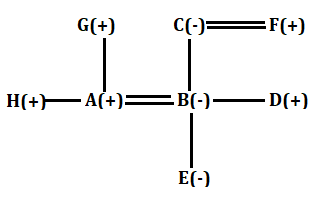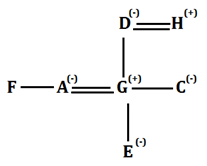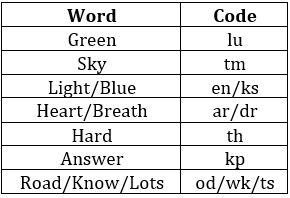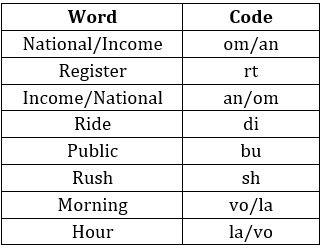Latest Banking jobs   »

# Reasoning Ability Quiz For IBPS SO Prelims 2022- 29th November

Direction (1-3): Study the following information carefully and answer the given questions.
There are eight members in a family i.e. A, B, C, D, E, F, G and H. There are three females and two married couples. A is son-in-law of C. B is daughter in law of G who is father of H. H is unmarried. D is son of C. C married to F who is grandfather of E. E is niece of H who is brother of A.

Q1. Who among the following is brother-in-law of D?
(a) G
(b) C
(c) A
(d) B
(e) None of these

Q2. Who among the following is brother-in-law of B?
(a) A
(b) H
(c) D
(d) G
(e) None of these

Q3. Who among the following is father of D?
(a) E
(b) F
(c) A
(d) H
(e) None of these

Directions (4-5): Read the following information carefully and answer the given questions.
There are eight people in a family, all of them are going for vacation. There are two married couple in the family. C is the mother of B. E is the daughter of A who is the sister of F. C is the only sister of G. H is father of C. D is the mother of C. A is the wife of G.

Q4. Who among the following is grandfather of E?
(a) B
(b) G
(c) C
(d) H
(e) None of these

Q5. How F is related to G?
(a) Sister-in-law
(b) Mother
(c) Son
(d) Brother
(e) Cannot be determine

Directions (6-10): Study the following information carefully and answer the questions given below:
In a certain code language:
‘Light Green Blue Sky’ is written as ‘en lu ks tm’,
‘Heart Sky Breath Hard’ is written as ‘tm ar th dr’,
‘Hard light Blue Answer’ is written as ‘th en kp ks’

Q6. What is the code for ‘Green’ in the given code language?
(a) En
(b) Lu
(c) Ks
(d) Tm
(e) None of these

Q7. What may be the code for ‘light Heart Sky’ in the given code language?
(a) en th tm
(b) lu ks dr
(c) en ar tm
(d) tm en ks
(e) None of these

Q8. What is the code for ‘know lots Road Hard’ in the given code language?
(a) od wk ts th
(b) od en wk ts
(c) kp dr tm od
(d) wk ts od ks
(e) None of these

Q9. What is the code for ‘Sky’ in the given code language?
(a) wk
(b) kp
(c) od
(d) ts
(e) None of these

Q10. What may be the code for ‘Answer Breath’?
(a) ks ar
(b) wk lu
(c) dr kp
(d) od en
(e) None of these

Directions (11-15): Study the following information carefully and answer the questions given below:
In a certain code language
‘National register Income’ is written as ‘an rt om’,
‘Ride Public Rush’ is written as ‘di bu sh’,
‘Morning Rush Hour’ is written as ‘la vo sh’,
‘Income Ride National’ is written as ‘om di an’.

Q11. What is the code for ‘Public’?
(a) di
(b) sh
(c) la
(d) bu
(e) None of these

Q12. Which of the following may be the code for ‘Avoid Rush Hour’?
(a) sh vo om
(b) vo sh an
(c) sh bl la
(d) Either (a) or (c)
(e) None of these

Q13. What is the code for ‘National’?
(a) rt
(b) an
(c) om
(d) vo
(e) Can’t be determined

Q14. What does ‘di’ stand for?
(a) ride
(b) national
(c) income
(d) rush
(e) None of these

Q15. What is the code for ‘Rush’?
(a) bu
(b) di
(c) sh
(d) vo
(e)None of these

Solutions

Solutions (1-3):
Sol.S1. Ans. (c)
S2. Ans. (b)
S3. Ans. (b)

Solutions (4-5):
Sol.S4. Ans. (d)
S5. Ans. (e)

Solution (6-10):
Sol.S6. Ans. (b)
S7. Ans. (c)
S8. Ans. (a)
S9. Ans. (e)
S10. Ans. (c)

Solutions (11-15):
Sol.S11. Ans. (d)
S12. Ans. (c)
S13. Ans. (e)
S14. Ans. (a)
S15. Ans. (c)#### Congratulations!Union Budget 2023-24: Free PDF